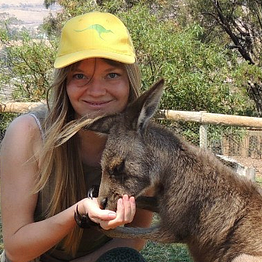# `Cowsay` 🐮

`import cowsaycowsay.cow('Hello everybody!')`

# Enchanced Tabular Data Visualization (Pandas)

`import pandas as pdimport numpy as npdf = pd.DataFrame(np.random.randn(20, 40))# Renaming columnsdf.columns = [x for x in 'abcdefghijklmnopqrstuvwxyzABCDEFGHIJKLMN']# Adding some missing values df.iloc[3,4] = np.nandf.iloc[2,0] = np.nandf.iloc[4,5] = np.nandf.iloc[0,6] = np.nandf.head()`

# Removing spines

`import numpy as npimport matplotlib.pyplot as pltx = np.arange(0, 5, 1)y = 2 * xplt.plot(x, y)plt.show()`

# Comparing Different Ways of Displaying Categorical Data in Python

`import pandas as pddct = {'Oceania': 8.5, 'Europe': 10, 'Antarctica': 14.2, 'South America': 17.8,        'North America': 24.2, 'Africa': 30.4, 'Asia': 44.6}continents = list(dct.keys())populations = list(dct.values())`

# 1. `is_unique`

`import pandas as pdprint(pd.Series([1, 2, 3, 4]).is_unique)print(pd.Series([1, 2, 3, 1]).is_unique)Output:TrueFalse`

# 2 & 3. `is_monotonic` and `is_monotonic_decreasing`

`print(pd.Series([1, 2, 3, 8]).is_monotonic)print(pd.Series([1, 2, 3, 1]).is_monotonic)print(pd.Series([9…`

# 2 Efficient Ways of Creating Fancy Pictogram Charts in Python